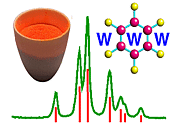Further Interpretations of Diffraction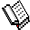Course Material Index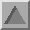Section Index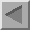Previous Page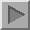Next Page

Further Interpretations of Diffraction

It is time now to bring together many observations on diffraction. We start with the three von Laue conditions (equations on previous page) which define when diffraction occurs. It so happens that these same three conditions together also define a geometrical plane in the crystal which intersects the three axes by amounts a/h, b/k and c/l respectively (see below, in the example given h = k = l = 2):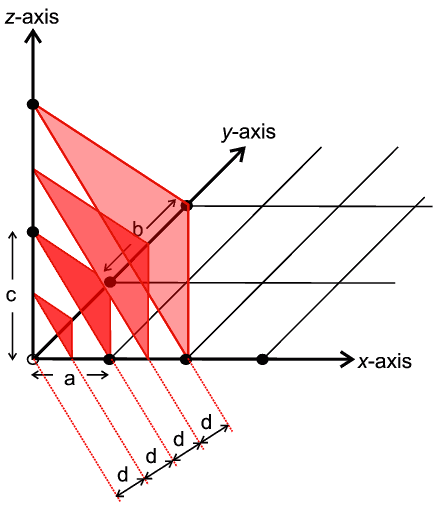Further this plane is at a distance d which is equal to 1/S from the origin O. We can imagine a whole set of parallel planes, as indicated in the Figure, in which each plane is separated from the next by d. We then say that such a set of planes are characterised by these three indices h,k,l. In fact the planes are invariably referred to as Bragg planes (after Bragg) and the indices as Miller indices (after such a system devised by Miller in 1839); the Miller indices often appear as a subscript, e.g. dhkl meaning the d spacing between the set of planes defined by h,k,l (for more notes on Miller indices you can click here).

However the planes h,k,l have further significance. Combining the knowledge that d = 1/S and that S = 2 sin θ/λ gives rise to the equation:

 λ = 2 d sin θ
which is almost identical to the famous Bragg's law:
 n λ = 2 d sin θ
Thus we now have a simple rigorous equation, that any student should be able to use to predict when diffraction will occur. The only difference between the two is that the lower one also caters for higher orders of diffraction (n>1) which is not so important here. We might also wonder whether there can be any further significance to the Miller planes (h,k,l) which so far only appear to be involved in diffraction by the mathematical accident that their equation happens to be equivalent to the (von Laue) conditions for diffraction. In fact a diagram which is well known to most science students (in two forms given below), usually accompanying the mention of Bragg's law, brings out another feature: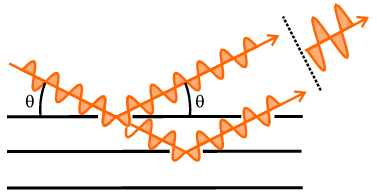From the geometry of either version in the above diagram, it can be easily shown that the difference in path length of two X-ray beams scattered from points in successive planes would amount to d sin θ. So another interpretation of Bragg's law is that it represents the condition that the path difference between these two X-ray beams equals a whole wavelength (or by higher multiples if n>1); of course we know that when two interfering waves differ in phase by exactly one wavelength, then they are effectively in-phase and will interfere constructively. This notion has clearly served crystallography well over the past 80-90 years with crystallographers strongly associating a given h,k,l diffraction with the h,k,l planes and their separation, d. This association has been strengthened further by the popular use of terms such as "reflection by a set of h,k,l planes" to describe diffraction under a h,k,l set of von Laue conditions. However there is a negative side to this balance sheet: many crystallography students have come away with the incorrect notion that X-rays are actually reflected by these Bragg h,k,l planes. This is of course nonsense, given that the planes are abstract mathematical items and therefore are not capable of "reflecting" anything; any student who has read this section should realise at least by now that diffraction is a product of the scattering of X-rays by atoms (with their electron clouds). It just so happens that this multiple atomic scattering process occurs at the same instant that projected path differences from Bragg planes equal a whole number of wavelengths. As a student of this course we hope you will be able to use the convenience of the notation of Bragg diffracting planes without believing that they really reflect: always remember, planes are virtual but atoms are real.Course Material IndexSection IndexPrevious PageNext Page
 © Copyright 1997-2006.  Birkbeck College, University of London. Author(s): Paul Barnes Simon Jacques Martin Vickers